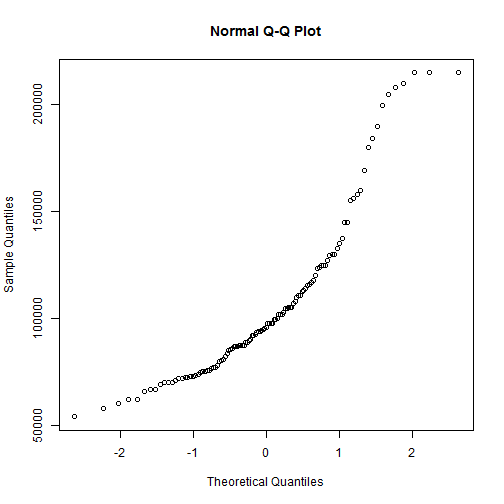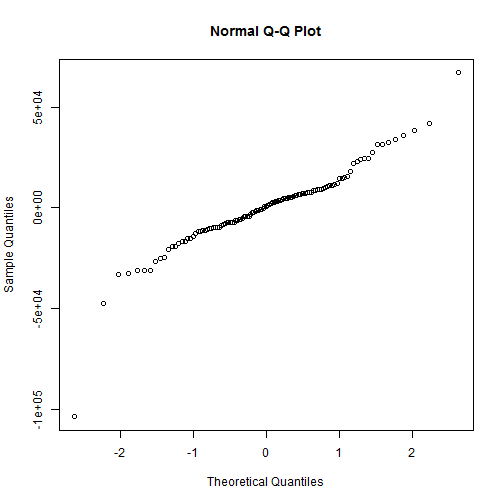# The qqplot

This program was written by Steve Simon, http://www.pmean.com, and is available at http://www.pmean.com/14/qqplot.html.

See a list of my other R programs at http://www.pmean.com/category/Rsoftware.html

Many statistical procedures are based on the assumption that your data has a normal distribution. The normal probability plot is a useful graphical tool for asessing this assumption. This plot is also called the qqplot (quantile-quantile plot).

You can use the housing data set to illustrate the use of the normal probability prlot.

``````al <- read.table(file="housing.txt",header=TRUE)
head(al)
``````
``````##    Price SquareFeet AgeYears NumberFeatures Northeast CustomBuild
## 1 205000       2650       13              7       Yes         Yes
## 2 208000       2600        *              4       Yes         Yes
## 3 215000       2664        6              5       Yes         Yes
## 4 215000       2921        3              6       Yes         Yes
## 5 199900       2580        4              4       Yes         Yes
## 6 190000       2580        4              4       Yes          No
##   CornerLot
## 1        No
## 2        No
## 3        No
## 4        No
## 5        No
## 6        No
``````
``````tail(al)
``````
``````##     Price SquareFeet AgeYears NumberFeatures Northeast CustomBuild
## 112 87400       1236        3              4        No          No
## 113 87200       1229        6              3        No          No
## 114 87000       1273        4              4        No          No
## 115 86900       1165        7              4        No          No
## 116 76600       1200        7              4        No          No
## 117 73900        970        4              4        No          No
##     CornerLot
## 112        No
## 113        No
## 114        No
## 115        No
## 116       Yes
## 117       Yes
``````
``````al\$age <- as.numeric(al\$AgeYears)
summary(al\$Price)
``````
``````##    Min. 1st Qu.  Median    Mean 3rd Qu.    Max.
##   54000   78000   96000  106000  120000  215000
``````
``````summary(al\$SquareFeet)
``````
``````##    Min. 1st Qu.  Median    Mean 3rd Qu.    Max.
##     837    1280    1550    1650    1890    3750
``````
``````summary(al\$age)
``````
``````##    Min. 1st Qu.  Median    Mean 3rd Qu.    Max.
##     1.0     1.0     5.0    10.5    20.0    31.0
``````
``````summary(al\$NumberFeatures)
``````
``````##    Min. 1st Qu.  Median    Mean 3rd Qu.    Max.
##    0.00    3.00    4.00    3.53    4.00    8.00
``````
``````summary(al\$Northeast)
``````
``````##  No Yes
##  39  78
``````
``````summary(al\$CustomBuild)
``````
``````##  No Yes
##  90  27
``````
``````summary(al\$CornerLot)
``````
``````##  No Yes
##  95  22
``````

The qqplot compares the data values to evenly spaced percentiles of the normal distribution. A straight line indicates that the normality assumption is reasonable. You should not over interpret minor deviations from linearity. A large deviation from linearity is an indication that the normality assumption may be questionable.

You can read more about this at http://www.pmean.com/09/NormalPlot.html

``````qqnorm(al\$Price)
``````In a linear model, the critical assumption is NOT that the predictor (independent) variables are normally distributed. It is NOT that the outcome (dependent) variable is normally distributed. It is that the residuals are normally distributed.

``````al.model <- lm(Price~SquareFeet+CustomBuild,data=al)
summary(al.model)
``````
``````##
## Call:
## lm(formula = Price ~ SquareFeet + CustomBuild, data = al)
##
## Residuals:
##     Min      1Q  Median      3Q     Max
## -103813   -9596     738    8784   67151
##
## Coefficients:
##                Estimate Std. Error t value Pr(>|t|)
## (Intercept)    11413.48    6549.92    1.74    0.084 .
## SquareFeet        55.36       4.12   13.43   <2e-16 ***
## CustomBuildYes 14285.99    5103.02    2.80    0.006 **
## ---
## Signif. codes:  0 '***' 0.001 '**' 0.01 '*' 0.05 '.' 0.1 ' ' 1
##
## Residual standard error: 19900 on 114 degrees of freedom
## Multiple R-squared:  0.732,  Adjusted R-squared:  0.727
## F-statistic:  156 on 2 and 114 DF,  p-value: <2e-16
``````
``````qqnorm(resid(al.model))
``````